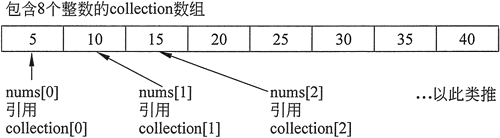## C++数组做函数参数详解

• 内容
• 评论
• 相关

```//This program demonstrates that an array element
//can be passed to a function like any other variable.
#include <iostream>
using namespace std;

void showValue(int); // Function prototype

int main()
{
const int ARRAY_SIZE = 8;
int collection[ARRAY_SIZE] = {5, 10, 15, 20, 25, 30, 35, 40};
for (int index = 0; index < ARRAY_SIZE; index++)
showValue(collection[index]);
cout << endl;
return 0;
}
void showValue(int num)
{
cout << num << " ";
}```

5 10 15 20 25 30 35 40

```void showValues (int nums[], int size)
{
for (int index = 0; index < size; index++)
cout << nums[index] << " ";
cout << endl;
}```

```// This program shows how to pass an entire array to a function.
#include <iostream>
using namespace std;

void showValues(int intArray[], int size); // Function prototype
int main()
{
const int ARRAY_SIZE = 8;
int collection[ARRAY_SIZE] = {5, 10, 15, 20, 25, 30, 35, 40};
cout << "The array contains the values\n";
showValues(collection, ARRAY_SIZE);
return 0;
}
void showValues (int nums[], int size)
{
for (int index = 0; index < size; index++)
cout << nums[index] << " ";
cout << endl;
}```

The array contains the values
5 10 15 20 25 30 35 40

void showValues(int []，int);

showValues(collection, ARRAY_SIZE);0条评论